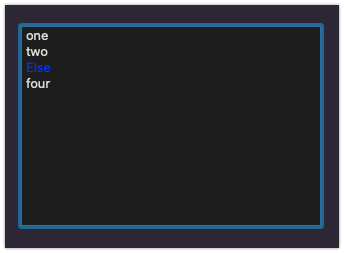# CellTextPaint not firing

I’m trying to change the color of the text of certain rows, but have had zero luck getting this to work. I’ve the documentation and read other posts in the forums, but it hasn’t helped any.

So, I’m just trying to get it to work at a basic level before moving on to more advanced things.

Here’s the basic setup I have:

In the CellTextPaint event I have:

``````cellName = me.cell(row, column)
if cellName = "Else" then
g.forecolor=RGB(0,0,255)
g.DrawString(Me.Cell(row, column), x, y)
end if
``````

and in the CellClick event I have:

``````me.invalidateCell(-1,-1)
``````

But the color of the text never turns blue when I click on that cell.

I’ve tried many variations of this, but obviously my brain is missing something crucial.

First If you are drawing the string yourself you need to return True so it is not overwritten by the framework.

Second If all you want to do is change the text color then all you need to do is omit the DrawString, then returning false as the framework will draw it in the forecolor you set… and the the whole code becomes:

``````if me.cell(row, column) = "Else" then
g.forecolor=RGB(0,0,255)
end if
``````

-Karen

1 Like

Doesn’t work. I tried it with and without returning False.

``````if me.cell(row, column) = "Else" then
g.forecolor=RGB(0,0,255)
Return False
end if
``````

You need to have Return True in the code in the CellTextPaint event. That tells the framework you are handling the text drawing and color yourself. Return False means nothing gets changed.

``````cellName = me.cell(row, column)
if cellName = "Else" then
g.forecolor=RGB(0,0,255)
g.DrawString(Me.Cell(row, column), x, y)
Return True
end if

``````

What version of Xojo are you using?

Using Xojo2023r1.1 I get this:with this code:

``````If Me.CellTextAt(row, column) = "Else" Then
g.forecolor=RGB(0,0,255)
End If
``````

in PaintCellText

Edit: the above is with DesktopListbox, changing that to Listbox:Same thing. Is using

``````me.invalidateCell(-1,-1)
``````

in the CellClick event enough?

Can you share a sample project that reproduce your problem using Dropbox, OneDrive or Google Drive?

What happens if you use this code?

``````If Me.CellTextAt(row, column) = "Else" Then
g.forecolor=RGB(0,0,255)
else
g.forecolor=RGB(255,0,0)
End If
``````

do you get red text instead?
If so, its firing, but not finding the word “else”
Have you tried putting a breakpoint in to see if it gets there?

No change. The breakpoints don’t even activate.

Which brings me back to the question…How do I get this to fire?

Is

``````me.invalidateCell(-1,-1)
``````

enough in the CellClick event when I click on the cell to fire off the CellTextPaint event?

OK, so this code I had in the CellTextEvent is blocking the code we’re trying here.

If i comment it out, the code here works,

``````If row=DroppedRow Then
g.forecolor=RGB(255,255,255)
g.DrawString(Me.Cell(row, column), x, y)
Return True
Else
Return False
End If
``````

So I had to move this code after the Blue “Else” code!

I sometimes turn on the horizontal lines then turn them off again in code to force an invalidate.

But the list should paint properly as soon as it is displayed.
Are you changing the text in code after it has been displayed?

That’s why I asked for a sample project as “something else” is affecting CellTextPaint in your project.

Well, I figured it out before I could create a smaller project to send you.

The question is, why does the dropped row code need to be after? It has a closed end if loop.

`If row=DroppedRow`

That came out of nowhere.So far you only seemed to be wanting blue text if the cell contains “Else”

That’s a separate action when I drag the row over to change the text white so that’s it’s viewable while the row on the 2nd listbox is being highlighted.

I’m sorry I don’t fully understand.

Do you have this code there too?

``````If row=DroppedRow Then
g.forecolor=RGB(255,255,255)
g.DrawString(Me.Cell(row, column), x, y)
Return True
Else
Return False
End If
``````

If you do, anything after that code will not execute as you are using Return True/False

Yes. I moved it below the blue “Else” code and it works now.

Do you understand why the code after that is not working?